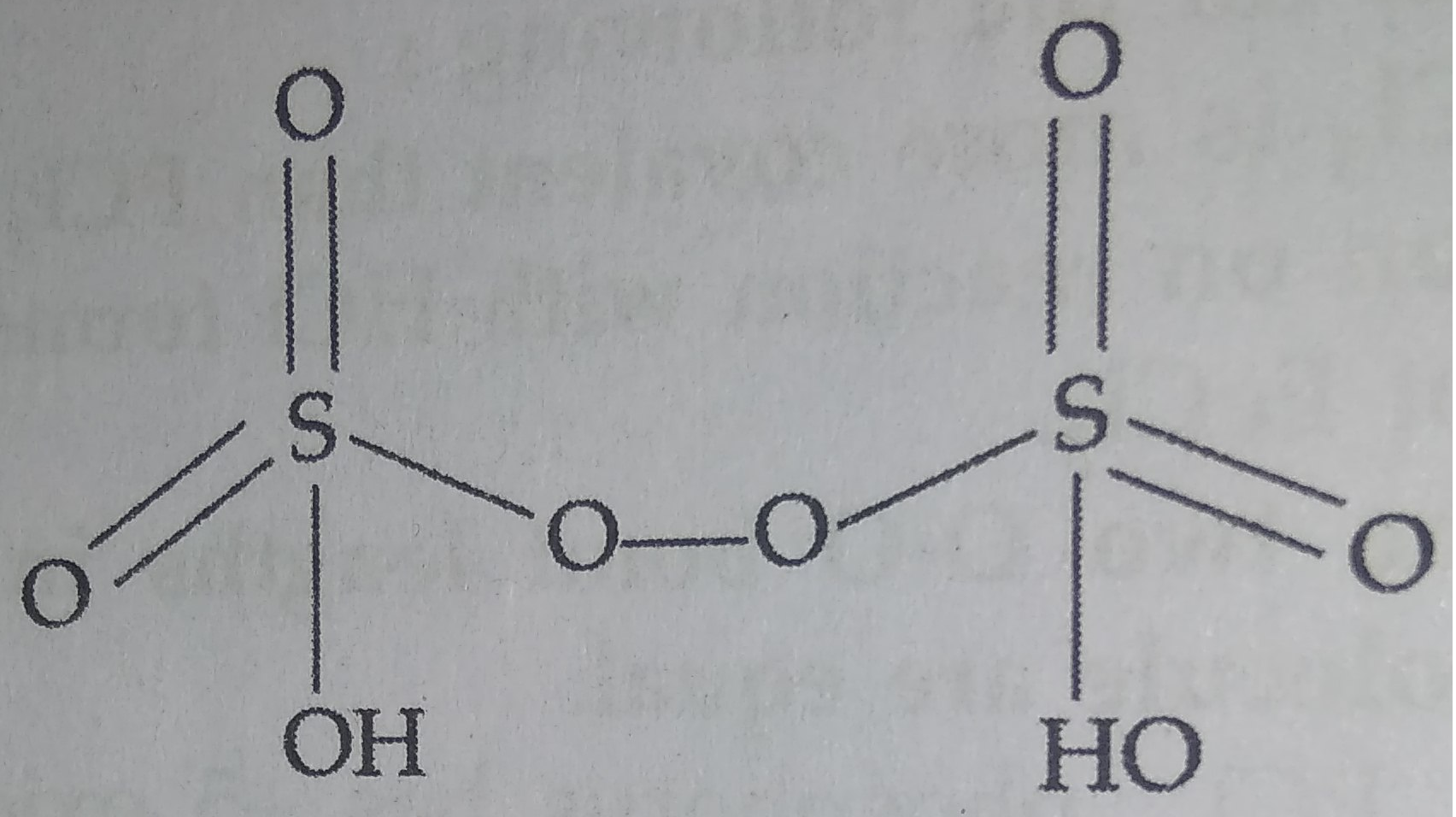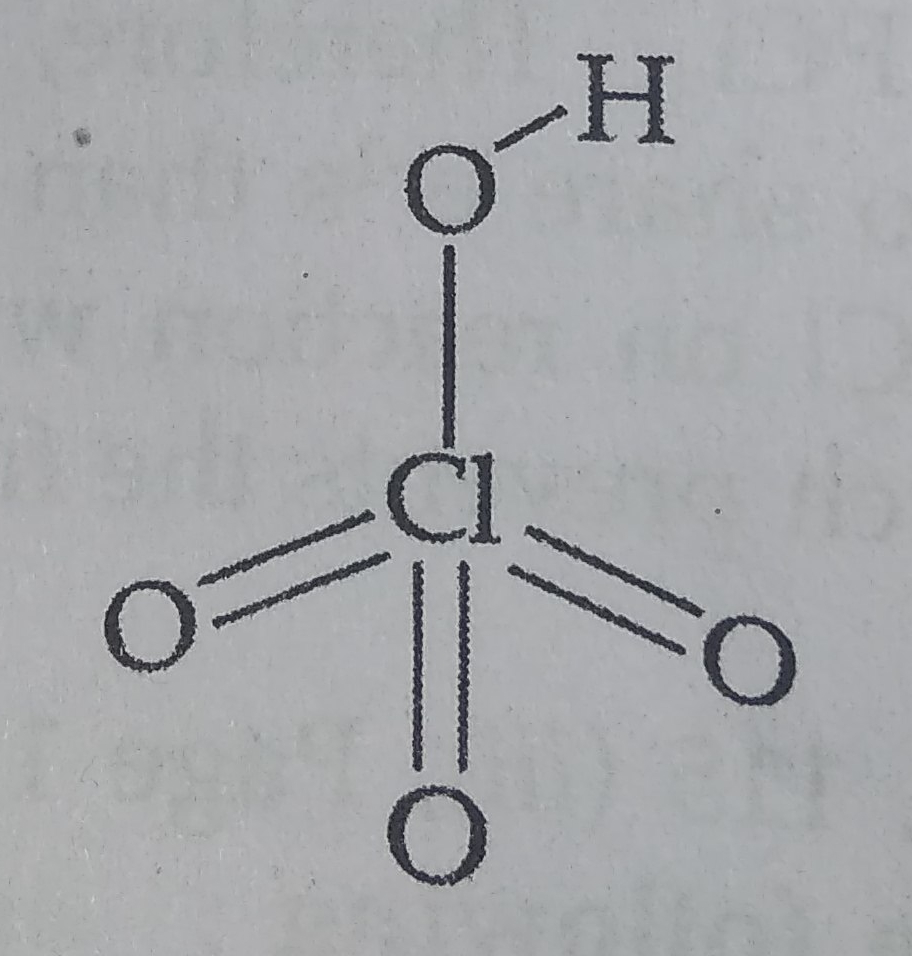(a) Draw the structures of the following:

(i) ${H}_{2}{S}_{2}{O}_{8}$                                     (ii) $HCl{O}_{4}$

(b) How would you account for the following:

(i) $N{H}_{3}$ is a stronger base than $P{H}_{3}.$

(ii) Sulphur has a greater tendency for catenation than oxygen.

(iii) ${F}_{2}$ is a stronger oxidising agent than $C{l}_{2}.$

Concept Videos :-

#7 | 17th Group

Concept Questions :-

group 17 ,preparation and properties

(a)  (i) ${H}_{2}{S}_{2}{O}_{8}$ (Peroxodisulphuric acid )or Marshall's acid :(ii) $HCl{O}_{4}$ (Perchloric acid) :(b) (i) Since both P and N contain lone pairs of electrons but due to small size and high electronegativity of Nitrogen in $N{H}_{3},$ the electron density is much higher than $P{H}_{3},$ therefore it can easily donate electrons and acts as strong Lewis base than $P{H}_{3}.$

(ii) The greater catenation tendency of sulphur is due to two reasons :

(a) The lone pair of electrons feels more repulsion in O-O bond than S-S bond due to its small size and thus S-S forms strong bond.

(b) As the size of atom increases down the group from O-PO, the strength of bond increases and therefore catenation tendency also increases.

(iii) Due to low bond dissociation enthalpy and high electronegativity of Fluorine, it has strong tendency to accept electrons and thus get reduced.

$F+{e}^{-}\to {F}^{-}$

Therefore ${F}_{2}$ acts as strong oxidising agent, while $C{l}_{2}$ is weak oxidising agent due to low electronegativity.

Difficulty Level: